# sklearn.preprocessing.MinMaxScaler¶

class sklearn.preprocessing.MinMaxScaler(feature_range=(0, 1), *, copy=True, clip=False)[source]

Transform features by scaling each feature to a given range.

This estimator scales and translates each feature individually such that it is in the given range on the training set, e.g. between zero and one.

The transformation is given by:

X_std = (X - X.min(axis=0)) / (X.max(axis=0) - X.min(axis=0))
X_scaled = X_std * (max - min) + min


where min, max = feature_range.

This transformation is often used as an alternative to zero mean, unit variance scaling.

Read more in the User Guide.

Parameters:
feature_rangetuple (min, max), default=(0, 1)

Desired range of transformed data.

copybool, default=True

Set to False to perform inplace row normalization and avoid a copy (if the input is already a numpy array).

clipbool, default=False

Set to True to clip transformed values of held-out data to provided feature range.

New in version 0.24.

Attributes:
min_ndarray of shape (n_features,)

Per feature adjustment for minimum. Equivalent to min - X.min(axis=0) * self.scale_

scale_ndarray of shape (n_features,)

Per feature relative scaling of the data. Equivalent to (max - min) / (X.max(axis=0) - X.min(axis=0))

New in version 0.17: scale_ attribute.

data_min_ndarray of shape (n_features,)

Per feature minimum seen in the data

New in version 0.17: data_min_

data_max_ndarray of shape (n_features,)

Per feature maximum seen in the data

New in version 0.17: data_max_

data_range_ndarray of shape (n_features,)

Per feature range (data_max_ - data_min_) seen in the data

New in version 0.17: data_range_

n_features_in_int

Number of features seen during fit.

New in version 0.24.

n_samples_seen_int

The number of samples processed by the estimator. It will be reset on new calls to fit, but increments across partial_fit calls.

feature_names_in_ndarray of shape (n_features_in_,)

Names of features seen during fit. Defined only when X has feature names that are all strings.

New in version 1.0.

minmax_scale

Equivalent function without the estimator API.

Notes

NaNs are treated as missing values: disregarded in fit, and maintained in transform.

For a comparison of the different scalers, transformers, and normalizers, see examples/preprocessing/plot_all_scaling.py.

Examples

>>> from sklearn.preprocessing import MinMaxScaler
>>> data = [[-1, 2], [-0.5, 6], [0, 10], [1, 18]]
>>> scaler = MinMaxScaler()
>>> print(scaler.fit(data))
MinMaxScaler()
>>> print(scaler.data_max_)
[ 1. 18.]
>>> print(scaler.transform(data))
[[0.   0.  ]
[0.25 0.25]
[0.5  0.5 ]
[1.   1.  ]]
>>> print(scaler.transform([[2, 2]]))
[[1.5 0. ]]


Methods

 fit(X[, y]) Compute the minimum and maximum to be used for later scaling. fit_transform(X[, y]) Fit to data, then transform it. get_feature_names_out([input_features]) Get output feature names for transformation. get_params([deep]) Get parameters for this estimator. Undo the scaling of X according to feature_range. partial_fit(X[, y]) Online computation of min and max on X for later scaling. set_output(*[, transform]) Set output container. set_params(**params) Set the parameters of this estimator. Scale features of X according to feature_range.
fit(X, y=None)[source]

Compute the minimum and maximum to be used for later scaling.

Parameters:
Xarray-like of shape (n_samples, n_features)

The data used to compute the per-feature minimum and maximum used for later scaling along the features axis.

yNone

Ignored.

Returns:
selfobject

Fitted scaler.

fit_transform(X, y=None, **fit_params)[source]

Fit to data, then transform it.

Fits transformer to X and y with optional parameters fit_params and returns a transformed version of X.

Parameters:
Xarray-like of shape (n_samples, n_features)

Input samples.

yarray-like of shape (n_samples,) or (n_samples, n_outputs), default=None

Target values (None for unsupervised transformations).

**fit_paramsdict

Returns:
X_newndarray array of shape (n_samples, n_features_new)

Transformed array.

get_feature_names_out(input_features=None)[source]

Get output feature names for transformation.

Parameters:
input_featuresarray-like of str or None, default=None

Input features.

• If input_features is None, then feature_names_in_ is used as feature names in. If feature_names_in_ is not defined, then the following input feature names are generated: ["x0", "x1", ..., "x(n_features_in_ - 1)"].

• If input_features is an array-like, then input_features must match feature_names_in_ if feature_names_in_ is defined.

Returns:
feature_names_outndarray of str objects

Same as input features.

get_params(deep=True)[source]

Get parameters for this estimator.

Parameters:
deepbool, default=True

If True, will return the parameters for this estimator and contained subobjects that are estimators.

Returns:
paramsdict

Parameter names mapped to their values.

inverse_transform(X)[source]

Undo the scaling of X according to feature_range.

Parameters:
Xarray-like of shape (n_samples, n_features)

Input data that will be transformed. It cannot be sparse.

Returns:
Xtndarray of shape (n_samples, n_features)

Transformed data.

partial_fit(X, y=None)[source]

Online computation of min and max on X for later scaling.

All of X is processed as a single batch. This is intended for cases when fit is not feasible due to very large number of n_samples or because X is read from a continuous stream.

Parameters:
Xarray-like of shape (n_samples, n_features)

The data used to compute the mean and standard deviation used for later scaling along the features axis.

yNone

Ignored.

Returns:
selfobject

Fitted scaler.

set_output(*, transform=None)[source]

Set output container.

See Introducing the set_output API for an example on how to use the API.

Parameters:
transform{“default”, “pandas”}, default=None

Configure output of transform and fit_transform.

• "default": Default output format of a transformer

• "pandas": DataFrame output

• None: Transform configuration is unchanged

Returns:
selfestimator instance

Estimator instance.

set_params(**params)[source]

Set the parameters of this estimator.

The method works on simple estimators as well as on nested objects (such as Pipeline). The latter have parameters of the form <component>__<parameter> so that it’s possible to update each component of a nested object.

Parameters:
**paramsdict

Estimator parameters.

Returns:
selfestimator instance

Estimator instance.

transform(X)[source]

Scale features of X according to feature_range.

Parameters:
Xarray-like of shape (n_samples, n_features)

Input data that will be transformed.

Returns:
Xtndarray of shape (n_samples, n_features)

Transformed data.

## Examples using sklearn.preprocessing.MinMaxScaler¶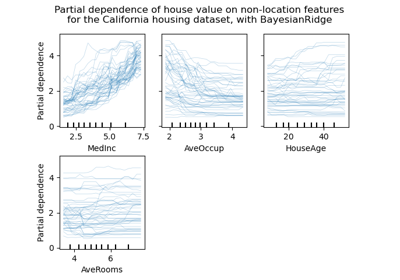Release Highlights for scikit-learn 0.24

Release Highlights for scikit-learn 0.24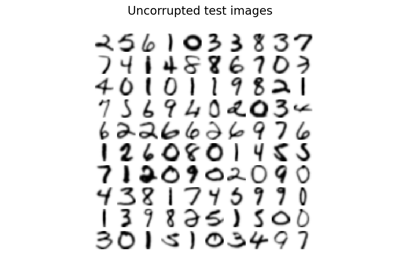Image denoising using kernel PCA

Image denoising using kernel PCA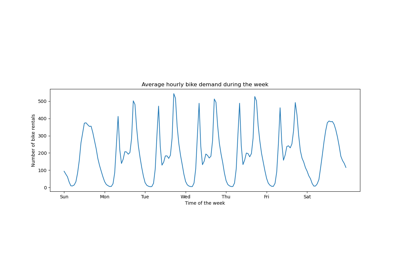Time-related feature engineering

Time-related feature engineering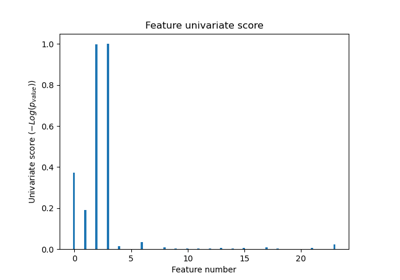Univariate Feature Selection

Univariate Feature Selection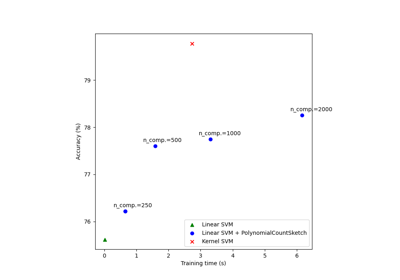Scalable learning with polynomial kernel approximation

Scalable learning with polynomial kernel approximation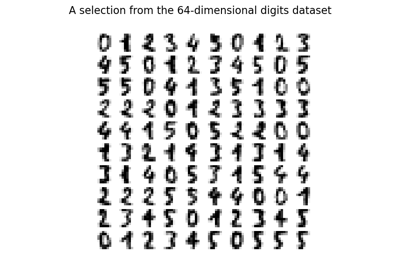Manifold learning on handwritten digits: Locally Linear Embedding, Isomap…

Manifold learning on handwritten digits: Locally Linear Embedding, Isomap...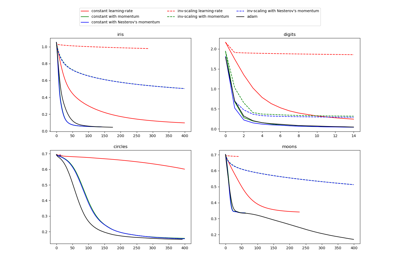Compare Stochastic learning strategies for MLPClassifier

Compare Stochastic learning strategies for MLPClassifier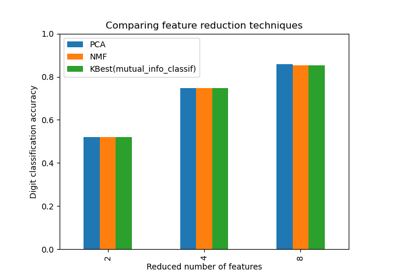Selecting dimensionality reduction with Pipeline and GridSearchCV

Selecting dimensionality reduction with Pipeline and GridSearchCV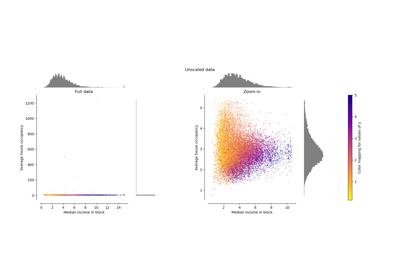Compare the effect of different scalers on data with outliers

Compare the effect of different scalers on data with outliers